Simple way of understanding a combination

In mathematics, a combination is a way of selecting items from a collection, such that (unlike permutations) the order of selection does not matter. (see Wikipedia)

For example:

We have a collection with the numbers 1 till 5 and we choose a subset of 3 numbers out of this collection. The order does not matter.

First we can choose out of 5 numbers: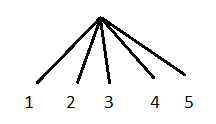After having chosen "1" we can choose "2", "3", "4" or "5":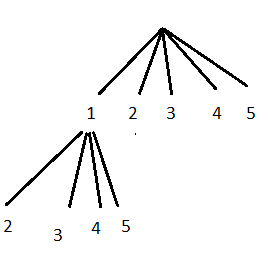After having chosen "1" and "2"  we can choose  "3", "4" or "5":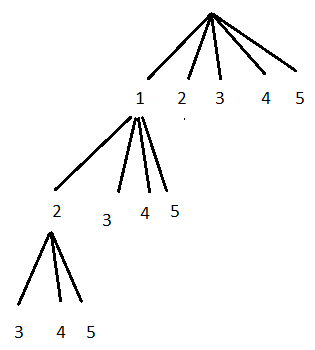So we can have [1,2,3], [1,2,4] or [1,2,5]

The total number of subsets is:

5 x 4 x 3 = 60  (this the way multiplying is explained in elementary school: 4 groups of 3 = 12  and 5 groups of 12 = 60 = 5x4x3)

But many of these subsets are the same, because the order does not matter:  [1,2,3],[1,3,2],  [2,1,3], [2,3,1]  [3,2,1], [3,1,2] ... These are called permutations.

The number of permutations of 3 elements is: 3 x 2 x 1= 6  (you could make a same tree diagram as above).

The total number of subsets: 60  = C  x 6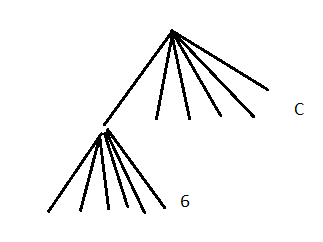We have:

6 of  [1,2,3],[1,3,2],  [2,1,3], [2,3,1]  [3,2,1], [3,1,2]

6 of  [4,2,3],[4,3,2],  [2,4,3], [2,3,4]  [3,2,4], [3,4,2]

6 of  [5,2,3],[5,3,2],  [2,5,3], [2,3,5]  [3,2,5], [3,5,2]

etc.

Totally we have C groups of 6 = C x 6

In the first branch we have all permutations of [1,2,3], in the second branch we have all permutations of [2,3,4] etc.

The amount of combinations is now C = 60 : 6 = 10   =  5x4x3 /  3x2x1   (dividing is the contrary of multiplying, as explained in elementary school..)

amount of combinations =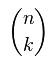=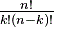=  5 x 4 x 3 / (3x2x1)  (in our example)

This is the way I understand the formula of combinations. I hope this explanation is useful for somebody. Anyway it is for myself, to refresh my memory sometimes in the future

Rinze Joustra